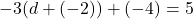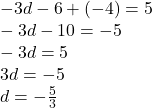## -3(d + -2) + -4 = 5 solve for d​

Question

-3(d + -2) + -4 = 5

solve for d​

in progress 0
7 months 2021-07-20T20:29:35+00:00 2 Answers 3 views 0

d=−1

Step-by-step explanation:

−3(d−2)−4=5

Step 1: Simplify both sides of the equation.

−3(d−2)−4=5

(−3)(d)+(−3)(−2)+−4=5(Distribute)

−3d+6+−4=5

(−3d)+(6+−4)=5(Combine Like Terms)

−3d+2=5

−3d+2=5

Step 2: Subtract 2 from both sides.

−3d+2−2=5−2

−3d=3

Step 3: Divide both sides by -3.

−3d

−3

=

3

−3

d=−1

2.